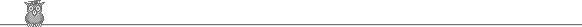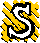### 53rd Putnam 1992A1.  Let Z be the integers. Prove that if f : Z → Z satisifies f( f(n) ) = f( f(n+2) + 2 ) = n for all n, and f(0) = 1, then f(n) = 1 - n.A2.  Let the coefficient of x1992 in the power series (1 + x)α = 1 + αx + ... be C(α). Find ∫01 C(-y-1) ∑k=119921/(y+k) dy.A3.  Find all positive integers a, b, m, n with m relatively prime to n such that (a2 + b2)m = (ab)n.A4.  Let R be the reals. Let f : R → R be an infinitely differentiable function such that f(1/n) = n2/(n2+1) for n = 1, 2, 3, ... Find the value of the derivatives of f at zero: f(k)(0) for k = 1, 2, 3, ... .A5.  Let N be the positive integers. Define f : N → {0, 1} by f(n) = 1 if the number of 1s in the binary representation of n is odd and 0 otherwise. Show that there do not exist positive integers k and m such that f(k + j) = f(k + m + j) = f(k + 2m + j) for 0 ≤ j < m.A6.  Four points are chosen independently and at random on the surface of a sphere (using the uniform distribution). What is the probability that the center of the sphere lies inside the resulting tetrahedron?B1.  Let R be the reals. Let S ⊆ R have n ≥ 2 elements. Let AS = { x ∈ R : x = (s + t)/2 for some s, t ∈ S with s ≠t}. What is the smallest possible |AS|?B2.  Show that the coefficient of xk in the expansion of (1 + x + x2 + x3)n is ∑j=0k  nCj   nC(k-2j).B3.  Let S be the set of points (x, y) in the plane such that the sequence an defined by a0 = x, an+1 = (an2 + y2)/2 converges. What is the area of S?B4.  p(x) is a polynomial of degree < 1992 such that p(0), p(1), p(-1) are all non-zero. The 1992th derivative of p(x)/(x3 - x) = f(x)/g(x) for polynomials f(x) and g(x). Find the smallest possible degree of f(x).B5.  Let An denote the n-1 x n-1 matrix (aij) with aij = i + 2 for i = j, and 1 otherwise. Is the sequence (det An)/n! bounded?B6.  Let M be a set of real n x n matrices such that: (1) 1 ∈ M; (2) if A, B ∈ M, then just one of AB, - AB is in M; (3) if A, B ∈ M, then either AB = BA or AB = -BA; (4) if 1 ≠ A ∈ M, then there is at least one B ∈ M such that AB = - BA. Prove that M contains at most n2 matrices.To avoid possible copyright problems, I have changed the wording, but not the substance of all the problems. The original text of the problems and the official solutions are in American Mathematical Monthly 100 (1993) 757.

Putnam home